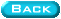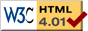Summary of resultsBack to my homepage   Research page        Results   Preprints   Personal info.   What's new For the definitions, please see the relevant pages, accessible through my research page. Closure operators on a subgroup lattice In joint work with Martha Kilpack (SUNY Oneonta), a characterization of the finite groups $G$ with the property that the closure operators on the subgroup lattice of $G$ form a lattice that is isomorphic to a subgroup lattice: this occurs if and only if $G$ is cyclic of prime power order This was extended in 2017 to closure operators on any finite lattice (the resulting lattice is a subgroup lattice if and only if the original lattice was a finite chain). In 2018, we completed the determination in the case of infinite groups as well; the resulting lattice is isomorphic to a subgroup lattice if and only if $G$ is either cyclic of prime power order, or isomorphic to the Prufer $p$-group $\mathbb{Z}_{p^{\infty}}$. Capability of groups A generalization of Baer's Theorem: Let k be a positive integer, and let $C_1,\ldots,C_n$, be cyclic $p$-groups, $p$ a prime with $p\gt k$, listed in ascending order of size. If $G$ is the $k$-nilpotent product of the $C_i$, then $G$ is capable if and only if $r\gt 1$ and the orders of the last two cyclic groups are equal. A necessary condition: Let $G$ be a $p$-group of class $k\gt 0$, and let $x_1,\ldots,x_r$ be a minimal generating set. Let the order of $x_i$ be $p^{a_i}$, with $a_1\leq\cdots\leq a_r$. If $G$ is capable, then $r\gt 1$ and $a_r\lt a_{r-1} + \lfloor(k-1)/(p-1)\rfloor$. The condition above is sufficient when $G$ is the $k$-nilpotent product of the cyclic groups generated by the $x_i$, and $p\leq k$. The inequality is best possible: for every $k$ and $p$ there is a capable example where we have equality. In the case of capable groups of class 2 and exponent $p$, with $p$ an odd prime, I have proven that given a group $G$, the capability of $G$ is equivalent to an explicit question about linear transformations between two finite dimensional vector spaces, and thus may be explicitly checked. Using this equivalence, I have obtained a number of necessary and of sufficient conditions for capability of $G$. A 5-generated $p$-group of class two and prime exponent is one and only one if nontrivial cyclic; a direct product amalgamating a cyclic subgroup of the commutators groups; or capable. A characterization of the capable 2-generated p-groups of class two. Dominions and amalgams in varieties of nilpotent groups A complete description of dominions in any variety all of whose elements are nilpotent groups of class at most 2. This appears in Amalgams of nilpotent groups of class 2, Journal of Algebra 274 (2004) pp. 1-63. There are nontrivial dominions in the variety of all nilpotent groups of class at most $k$ for any $k\gt 1$, and in the class of all nilpotent groups. Examples can be found in Dominions in varieties of nilpotent groups, Comm. Alg. 28 (2000) no. 3, pp. 1241-1270. A description of the absolutely closed nilpotent groups relative to any variety all of whose elements are nilpotent groups of class two. Again, this occurs in Amalgams of nilpotent groups of class two, Journal of Algebra 274 (2004) pp. 1-63. A complete characterisation of the weakly and strongly embeddable amalgams in any variety of nilpotent groups of class two, as well as a characterisation of the weak, strong, and special amalgamation bases and related results. Again, in Amalgams of nilpotent groups of class two. Dominions in the variety of metabelian groups There are nontrivial dominions in the variety of metabelian groups. In fact, there is a finitely generated group $G$, and a subgroup $H$ of $G$, which is abelian of finite rank, such that the dominion of $H$ in $G$ is abelian of infinite rank. The example can be found in Dominions in varieties of nilpotent groups, Comm. Alg. 28 (2000) no. 3, pp. 1241-1270. There are nontrivial dominions in the variety of all groups which are extensions of an abelian group of exponent $n$ by an abelian group of exponent $m$ (unpublished). Dominions in varieties generated by simple groups By generalizing an argument of B.H. Neumann, we fully characterize the dominions of subgroups of a finite nonabelian simple group $S$, in the variety generated by $S$. This characterization is used to provide many examples of varieties of groups with nonsurjective epimorphisms. They can be found in Dominions in varieties generated by simple groups, Alg. Universalis 48 (2002) pp. 133-143. Behavior of dominions under varietal multiplication If $\mathfrak{N}$ is a nontrivial variety which has instances of nontrivial dominions, and $\mathfrak{Q}$ is any variety other than the variety of all groups, then the varietal product $\mathfrak{NQ}$ also has nontrivial dominions. The converse of this is false, as witnessed by the fact, mentioned above, that the variety of metabelian groups, which is the product of the variety of abelian groups with itself, has nontrivial dominions, even though all dominions in the variety of abelian groups are trivial. In consequence, the variety of all solvable groups of length at most $k$ has nontrivial dominions, as does any product of abelian varieties with at least two factors. We give a lower and upper bound on the dominion of a subgroup $H$ of a group $G$ in the product variety $\mathfrak{NQ}$, in terms of the laws of $\mathfrak{Q}$, the dominions in $\mathfrak{N}$, and the internal structure of $G$. Under mild extra conditions on $G$, we can describe the dominion exactly. All of the above results can be found in Dominions in decomposable varieties, Alg. Unversalis 43 no. 2-3 (2000), pp. 217-232. Nonsurjective epimorphisms If a varietal product $\mathfrak{NQ}$ has nonsurjective epimorphisms, then so does $\mathfrak{N}$. In fact, the subgroup $H$ is epimorphically embedded into $G$ in the variety $\mathfrak{NQ}$ if and only if for every normal subgroup $M$ of $G$ such that $M\\in \mathfrak{N}$ and $G/M\in \mathfrak{Q}$, $H\cap M$ is epimorphically embedded into $M$ (in the variety $\mathfrak{N}$) and $HM=G$. If $H$ is epimorphically embedded into a finite nonabelian simple group $G$ in the variety $\mathfrak{N}$, and $\mathfrak{Q}$ is any variety other than the variety of all groups, then $\mathfrak{NQ}$ also has nonsurjective epimorphisms. If $H$ is epimorphically embedded into a finite group $G$ in the variety $\mathfrak{N}$, and $\mathfrak{Q}$ is a finite varietal product of nilpotent varieties, each of which contains the infinte cyclic group, then $\mathfrak{NQ}$ has instances of nonsurjective epimorphisms. The above results may be found in Nonsurjective epimorphisms in decomposable varieties, Alg. Universalis 48 (2002) pp. 145-150.avm1260@louisiana.edu